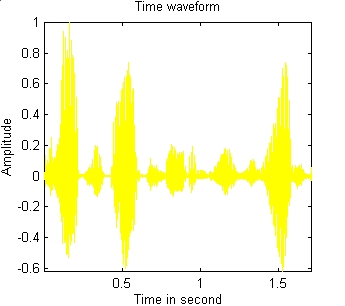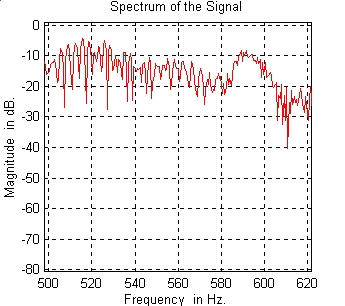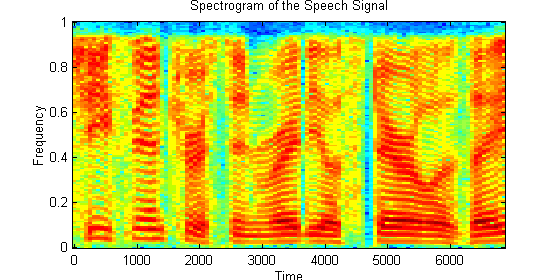Time and Frequency Representations Overview

In this experiment you learn about three representations of discrete-time signals. The first one is the standard time representation where the values of a sequence are plotted against time instants.  The  second representation is the
spectrum of  a sequence. This is taken to be to the logarithmic plot of the magnitude of the DFT of the sequence (the plot is normalized to have a maximum at 0 dB). The spectrum provides information about the frequency content of the sequence. The third representation is the spectrogram of a sequence. It provides information about how the frequency content of  the sequence changes with time; it is a plot of frequency against time with color used to indicate the relative strengths of the varied frequency components (warm colors indicate high amplitudes).

The experiment allows the user to choose from a selection of sounds:

1)  Bird chirps
2) Dropping egg
3) Train whistle
4) Beats
5) FM

All sequences have been obtained by sampling the original continuous-time signal at 8192Hz.
For each choice of sound, the user can plot the time sequence, its spectrum, or its spectrogram.

Here is an example.

• This is a time representation of a sentence. You can  listen to it.

•• This is a portion of the spectrum of the above speech signal between 500 and 620 Hz.• This is a plot of a typical the spectrogram of a speech signal. The spectrogram is a plot of the frequency content of the signal as a function of time. It is determined by computing the spectra of successive subintervals of the sequence and by plotting their magnitude using a color code. You can listen to the speech signal whose spectrogram  is shown below.
•